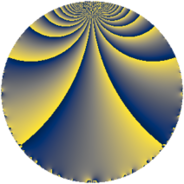# Properties

 Label 35.2.jLevel $35$ Weight $2$ Character orbit 35.j Rep. character $\chi_{35}(4,\cdot)$ Character field $\Q(\zeta_{6})$ Dimension $4$ Newform subspaces $1$ Sturm bound $8$ Trace bound $0$

# Related objects

## Defining parameters

 Level: $$N$$ $$=$$ $$35 = 5 \cdot 7$$ Weight: $$k$$ $$=$$ $$2$$ Character orbit: $$[\chi]$$ $$=$$ 35.j (of order $$6$$ and degree $$2$$) Character conductor: $$\operatorname{cond}(\chi)$$ $$=$$ $$35$$ Character field: $$\Q(\zeta_{6})$$ Newform subspaces: $$1$$ Sturm bound: $$8$$ Trace bound: $$0$$

## Dimensions

The following table gives the dimensions of various subspaces of $$M_{2}(35, [\chi])$$.

Total New Old
Modular forms 12 12 0
Cusp forms 4 4 0
Eisenstein series 8 8 0

## Trace form

 $$4 q - 2 q^{4} - 2 q^{5} - 4 q^{6} - 4 q^{9} + O(q^{10})$$ $$4 q - 2 q^{4} - 2 q^{5} - 4 q^{6} - 4 q^{9} - 4 q^{10} + 8 q^{14} + 8 q^{15} + 2 q^{16} + 12 q^{19} + 4 q^{20} - 10 q^{21} + 6 q^{24} + 6 q^{25} - 4 q^{26} - 28 q^{29} + 2 q^{30} - 4 q^{31} - 8 q^{34} - 16 q^{35} + 8 q^{36} - 4 q^{39} - 12 q^{40} + 20 q^{41} - 4 q^{45} + 6 q^{46} + 26 q^{49} + 16 q^{50} + 4 q^{51} + 10 q^{54} + 6 q^{56} + 20 q^{59} - 4 q^{60} - 14 q^{61} - 28 q^{64} + 8 q^{65} - 12 q^{69} - 10 q^{70} - 8 q^{71} - 16 q^{74} - 8 q^{75} - 24 q^{76} - 4 q^{79} + 2 q^{80} - 2 q^{81} + 8 q^{84} + 16 q^{85} - 14 q^{86} + 18 q^{89} + 16 q^{90} - 4 q^{91} + 12 q^{95} + 10 q^{96} + O(q^{100})$$

## Decomposition of $$S_{2}^{\mathrm{new}}(35, [\chi])$$ into newform subspaces

Label Dim $A$ Field CM Traces $q$-expansion
$a_{2}$ $a_{3}$ $a_{5}$ $a_{7}$
35.2.j.a $4$ $0.279$ $$\Q(\zeta_{12})$$ None $$0$$ $$0$$ $$-2$$ $$0$$ $$q+\zeta_{12}q^{2}+(-\zeta_{12}+\zeta_{12}^{3})q^{3}-\zeta_{12}^{2}q^{4}+\cdots$$